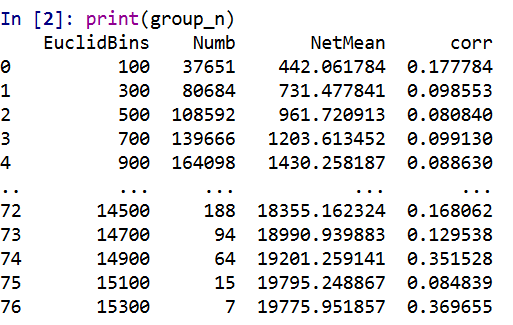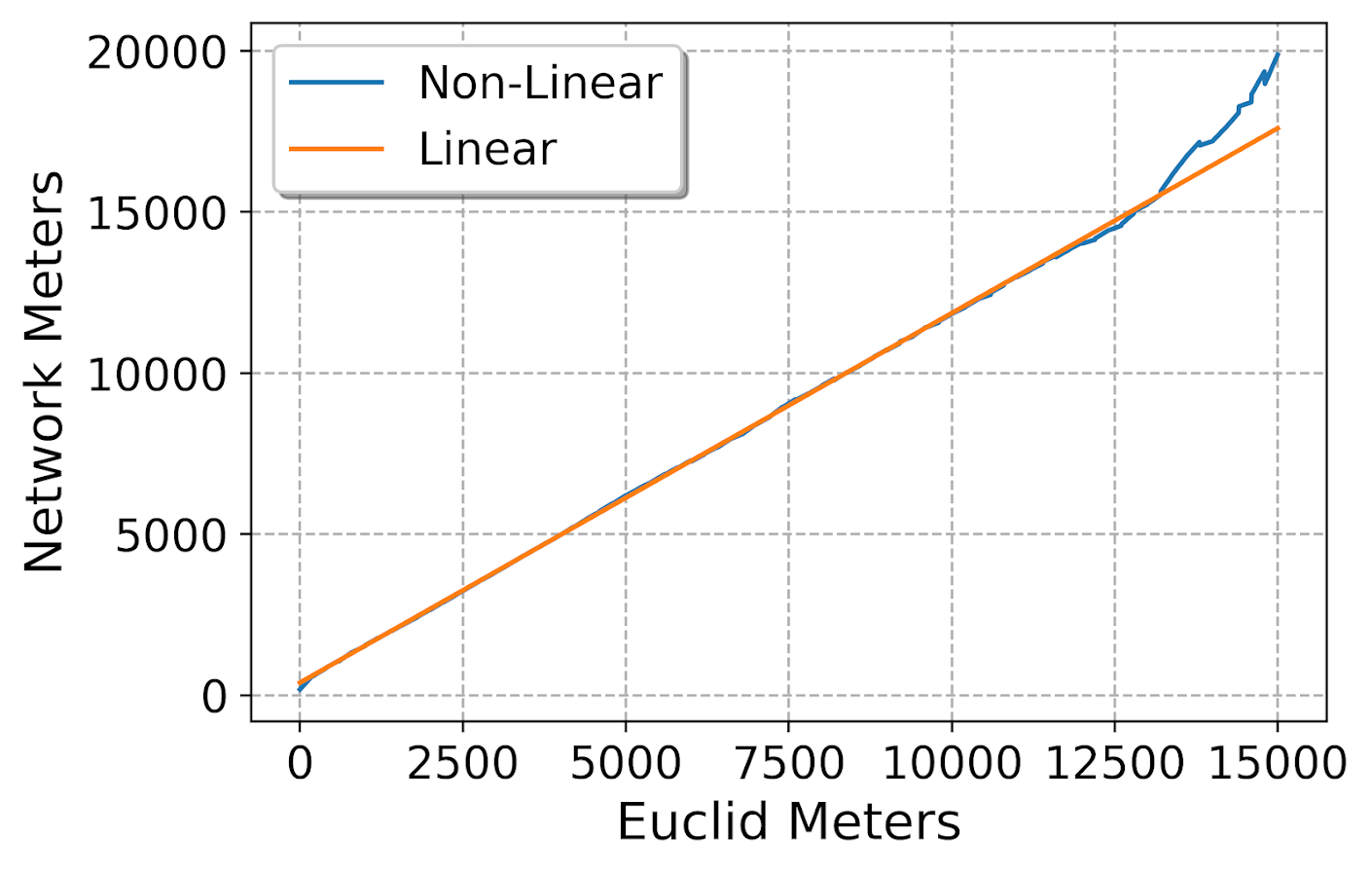# Street Network Distances and Correlations

Wouter Steenbeek (a friend and co-author for a few articles) has a few recent blog posts replicating some of my prior work replicating some of my work on street network vs Euclidean distances in Albany, NY (Wouters, 1, 2) and my posts (1,2).

In Wouter’s second post, he was particularly interested in checking out shorter distances (as that is what we are often interested in in criminology, checking crime clustering). When doing that, the relationship between network and Euclidean distances sometimes appear less strong, so my initial statement that they tend to be highly correlated is incorrect.

But this is an artifact for the correlation between any two measures – worth pointing out in general for analysis. If you artificially restrict the domain of one variable the correlation always goes down. See some examples on the cross-validated site (1, 2) that illustrate this with nicer graphs than I can whip up in a short time.

But for a quick idea about the issue, imagine a scenario where you slice out Euclidean distances in some X bin width, and check the scatterplot between Euclidean and network distances. So you will get less variation on the X axis, and more variation on the Y axis. Now take this to the extreme, and slice on Euclidean distances at only one value, say 100 meters exactly. In this scatterplot, there is no X variation, it is just a vertical line of points. So in that scenario the correlation is 0.

So I should not say the correlation between the two measures is high, as this is not always true – you can construct an artificial sample in which that statement is false. So a more accurate statement is that you can use the Euclidean distance to predict the network distance fairly accurately, or that the linear relationship between Euclidean and network distances is quite regular – no matter what the Euclidean distance is.

My analysis I have posted the python code here. But for a quick rundown, I grab the street networks for a buffer around Albany, NY using the osmnx library (so it is open street map network data). I convert this street network to an undirected graph (so no worrying about one-way streets) in a local projection. Then using all of the intersections in Albany (a few over 4000), I calculate all of the pairwise distances (around 8.7 million pairs, takes my computer alittle over a day to crunch it out in the background).

So again, the overall correlation is quite high:But if you chunk the data up into tinier intervals, here 200 meter intervals, the correlations are smaller (an index of 100 means `[0-200)`, 300 means `[200-400)`, etc.).But this does not mean the linear relationship between the two change. Here is a comparison of the linear regression line for the whole sample (orange), vs a broken-stick type model (the blue line). Imagine you take a slice of data, e.g. all Euclidean distances in the bin `[100-200)` and fit a regression line. And then do the same for the Euclidean distances `[200-300)` etc. The blue line here are those regression fits for each of those individual binned estimates. You can see that the two estimates are almost indistinguishable, so the relationship doesn’t change if you subset the data to shorter distances.Technically the way I have drawn the blue line is misleading, I should have breaks in the line (it is not forced to be connected between bins, like my post on restricted cubic splines is). But I am too lazy to write code to do those splits at the moment.

Now, what does this mean exactly? So for research designs that may want to use network distances and an independent variable, e.g. look at prison visitation as a function of distance, or in my work on patrol redistricting I had to impute some missing travel time distances, these are likely OK to use typical Euclidean distances. Even my paper on survivability for gun shot fatality shows improved accuracy estimates using network distances, but very similar overall effects compared to using Euclidean distances.

So while here I have my computer crunch out the network distances for a day, where the Euclidean distances with the same data only takes a second, e.g. using `scipy.spatial.distance`. So it depends on the nature of the analysis whether that extra effort is worth it. (It helps to have good libraries ease the work, like here I used osmnx for python, and Wouter showed R code using sf to deal with the street networks, hardest part is the networks are often not stored in a way that makes doing the routing very easy. Neither of those libraries were available back in 2014.) Also note you only need to do the network calculations once and then can cache them (and I could have made these network computations go faster if I parallelized the lookup). So it is slightly onerous to do the network computations, but not impossible.

So where might it make a difference? One common use of these network distances in criminology is for analyses like Ripley’s K or near-repeat patterns. I don’t believe using network distances makes a big deal here, but I cannot say for sure. What I believe happens is that using network distances will dilate the distances, e.g. if you conclude two point patterns are clustered starting at 100 meters using Euclidean distances, then if using network it may spread out further and not show clustering until 200 meters. I do not think it would change overall inferences, such as where you make an inference whether two point patterns are clustered or not. (One point is does make a difference is doing spatial permutations in Ripley’s K, you should definitely restrict the simulations to generating hypothetical distributions on the street network and not anywhere in the study area.)

Also Stijn Ruiter makes the point (noted in Wouter’s second post), that street networks may be preferable for prediction purposes. Stijn’s point is related to spatial units of analyses, not to Euclidean vs Network distances. You could have a raster spatial unit of analysis but incorporate street network statistics, and vice-versa could have a vector street unit spatial unit of analysis and use Euclidean distance measures for different measures related to those vector units.

Wouter’s post also brought up another idea I’ve had for awhile, that when using spatial buffers around areas they can be bad control areas, as even if you normalize the area they have a very tiny sliver of network distance attributable to them. I will need to show that for another blog post though. (This was mostly my excuse to learn osmnx to do the routing!)